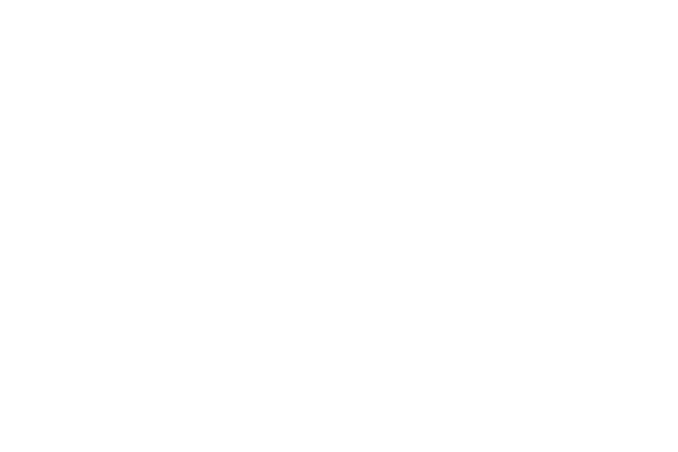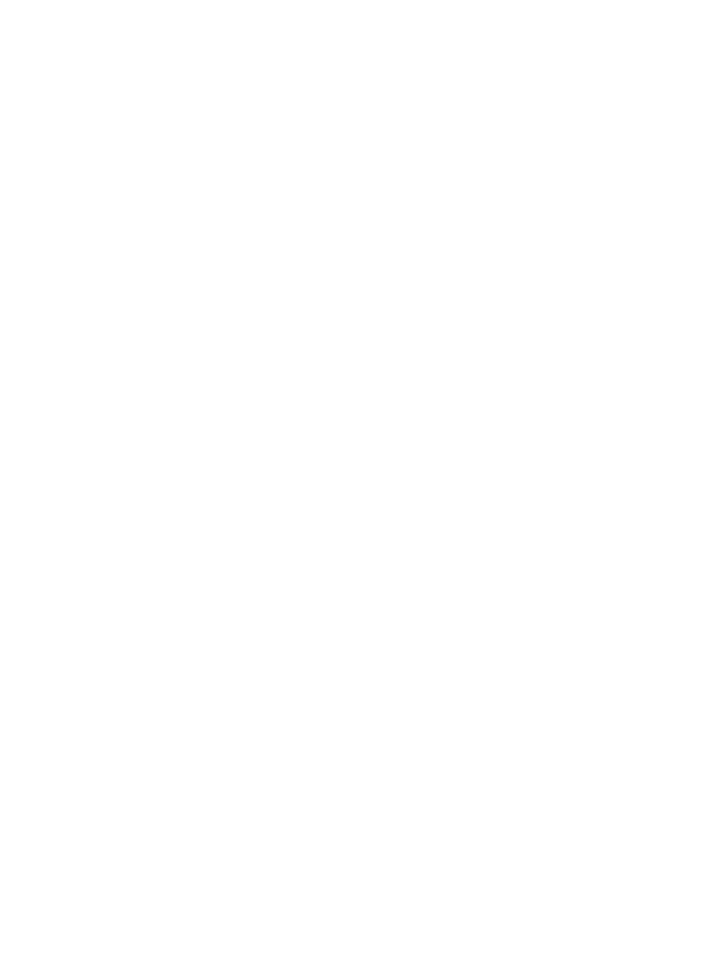# CBSE Rules for Basic Maths and STD. Maths or Applied Maths in Class 10th or 11th 2022

## CBSE Rules for Basic Maths and STD. Maths or Applied Maths in Class 10th or 11th 2022

CENTRAL BOARD OF SECONDARY EDUCATION
(An Autonomous Organisation under the Ministry of Education, Govt. of India)
CBSE/CE/2022/

To
The Principal
Schools Affiliated to CBSE
CIRCULAR
Date: 08.08.2022

Sub:- Permission to offer Mathematics (041) in Class-XI to the students who
offered Mathematics Basic (241) in Class-10

You are aware that session 2022 has also been affected severely by Covid and
the session has already been delayed, now there is a need for the remaining activities
of this session to be completed on time.

As per the earlier circular, students of Class-X who have studied Mathematics
Standard (041) can offer Mathematics (041) in Class-XI. In case, the students who
offered Mathematics Basic (241) in Class-X are allowed to offer only Applied
Mathematics in Class-XI.

This rule was relaxed by the CBSE in the past 2 years because of the circumstances developed due to the pandemic and thus, students who offered Mathematics Basic(241) were also allowed to offer Mathematics (041) in class XI.

As the current session has also been adversely affected by Covid, hence, it has
been decided to further extend this relaxation for one year i.e. 2022-23.

Before permitting Mathematics (041) in Class-XI to such students, the Head of
the Institution should satisfy that the students are having the aptitude and ability to pursue Mathematics (041) in Class-XI.

This exemption is being given as a special measure to facilitate the present
batch i.e., 2022-2023 of students of Class-XI.

## Official Circular of CBSE Rules for Basic Maths and STD. Maths or Applied Maths in Class 10th or 11th 2022CBSE Rules for Basic Maths and STD. Maths or Applied Maths in Class 10th or 11th 2022

## If you have any doubt regarding basic maths, Standard maths or Applied maths of class 9th, 10th, 11th & 12th. You can write in the comment box below.

Your queries of CBSE Rules for Basic Maths and STD. Maths or Applied Maths in Class 10th or 11th 2022 are most welcomed for our side.

CBSE Basic, Standard, Applied Maths Rule 2022-23

• CBSE Basic Maths 2022
• CBSE Standard Maths 2022 Rules
• CBSE Applied Maths Rules 2022CBSE Rules for Basic Maths and STD. Maths or Applied Maths in Class 10th or 11th 2022
• CBSE Basic and Standard Maths circular 2022
• Basic and Standard Maths in Class 10
• Is Standard Maths hard
• What is Basic Maths for Class 10
• Difference between basic and standard maths
• Basic maths Class 10 book
• Difference between Basic and Standard Maths in Class 10

## Can I opt for maths in class 11th if i had basic maths in 10th class?

No you can’t, you should go for std maths but in 2022-23 you can opt for maths in class 11th.

## Is basic math hard in class 9th and 10th?

Yes the comparison of std maths basic maths is easy.

## Is standard maths hard in class 9th and 10th?

Yes as a comparison of basic maths, standard maths is hard.

## Is applied maths the same as maths?

No, applied maths is easy rather than maths in class 11th and 12th.

## How can I opt applied maths in class 11th?

You can opt applied maths as a fifth subject in class 11th as an option in IP/PHE/Psychology/Applied Maths.

## Does the syllabus of basic maths and std maths syllabus be the same?

No both syllabus are different.